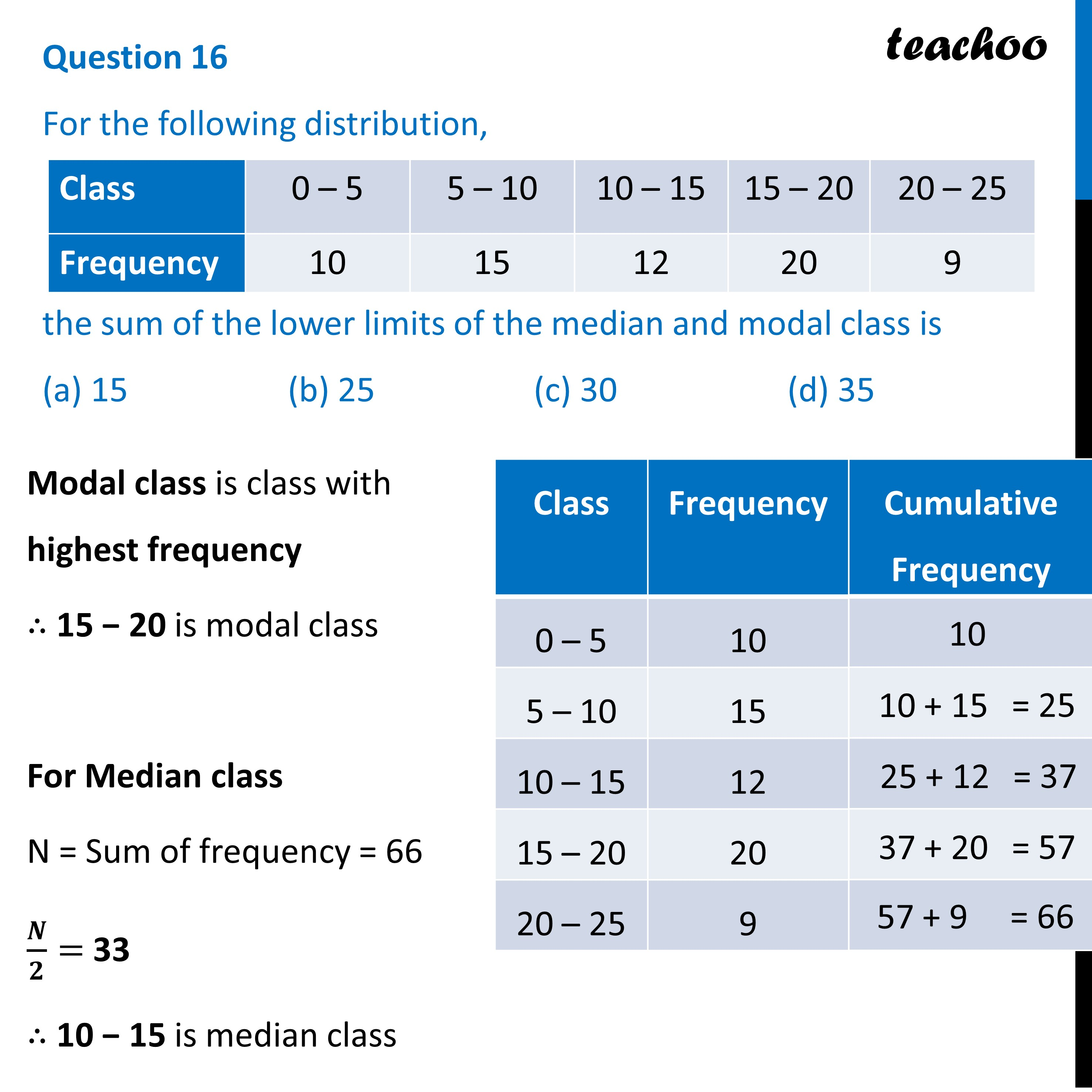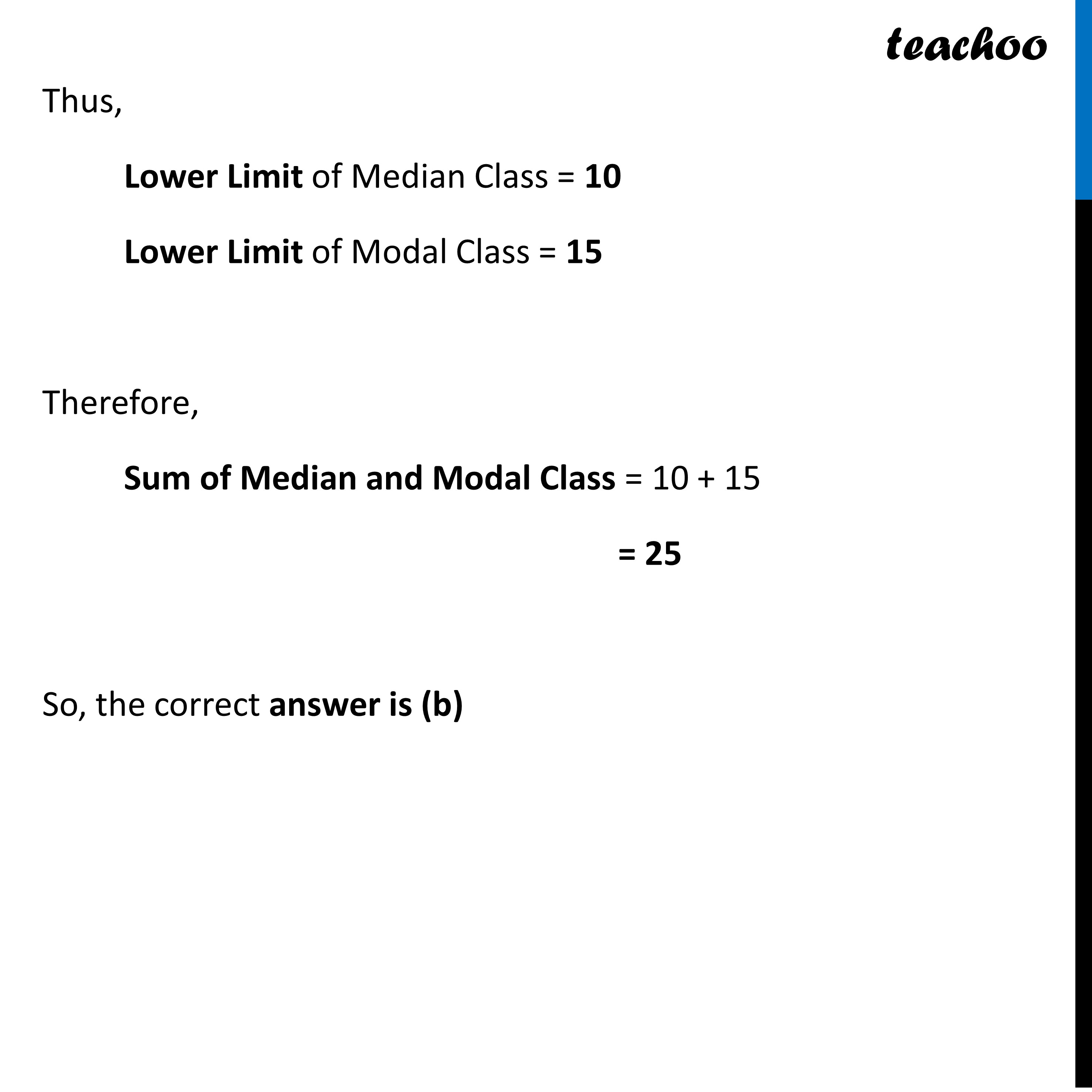CBSE Class 10 Sample Paper for 2023 Boards - Maths Standard

Class 10
Solutions of Sample Papers for Class 10 Boards

## For the following distribution,

 Class 0 – 5 5 – 10 10 – 15 15 – 20 20 – 25 Frequency 10 15 12 20 9

## (a) 15   (b) 25   (c) 30  (d) 35Learn in your speed, with individual attention - Teachoo Maths 1-on-1 Class

### Transcript

Question 16 For the following distribution, the sum of the lower limits of the median and modal class is (a) 15 (b) 25 (c) 30 (d) 35 Modal class is class with highest frequency ∴ 15 − 20 is modal class For Median class N = Sum of frequency = 66 𝑵/𝟐= 33 ∴ 10 − 15 is median class Thus, Lower Limit of Median Class = 10 Lower Limit of Modal Class = 15 Therefore, Sum of Median and Modal Class = 10 + 15 = 25 So, the correct answer is (b)